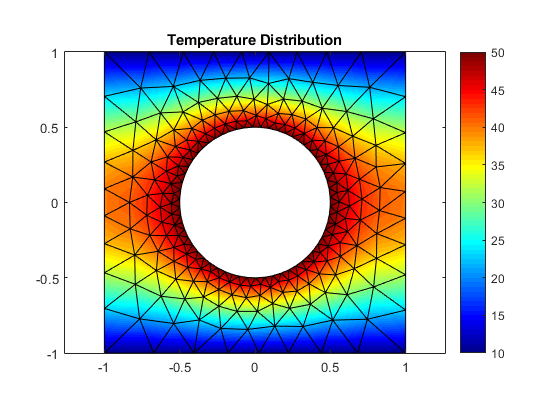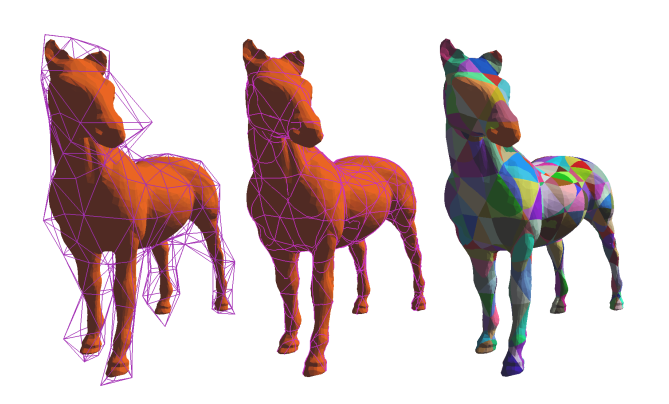# FINITE ELEMENT COURSE

This post is an introductory course to the Finite Element Method  (FEM). It corresponds to a one semester second undergrad course that we teach at the ETSEIB (UPC_BarcelonaTech).

It is a Matlab based course, where all the algorithms corresponding to 1D and 2D FEM are explained and implemented in Matlab. We also add many extra plot functions in order to obtain nice visualizations of the numerical computations (follow the link on the image below to get all the materials).The material is divided on Theory presentations and  the associated Practices developed using the methodology explained in our previous post  about to make a script (Com crear un guió de pràctiques).

Moreover, we are also interested in not only industrial but also computer animation applications of FEM to deformable models (follow the link on the image below to learn more).Impactes: 15174